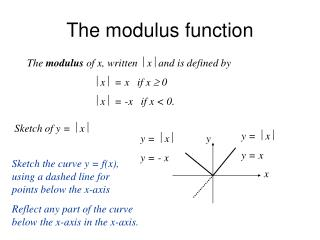DownloadDownload PresentationThe modulus function

# The modulus function

Download Presentation## The modulus function

- - - - - - - - - - - - - - - - - - - - - - - - - - - E N D - - - - - - - - - - - - - - - - - - - - - - - - - - -
##### Presentation Transcript

1. y x The modulus function The modulus of x, written  x and is defined by  x = x if x  0  x = -x if x < 0. Sketch of y =  x y =  x y = x y =  x y = - x Sketch the curve y = f(x), using a dashed line for points below the x-axis Reflect any part of the curve below the x-axis in the x-axis.

2. y x Sketch y =  2x - 1 The graph of y =  2x - 1 has the right-hand branch with equation y = 2x – 1. The left-hand branch is the negative of 2x – 1, i.e. y = -2x + 1. y = 2x - 1 y = -2x +1

3. Sketch y = cos x y = cos x x

4. Equations involving modulus Solve the equation 2x - 1 = 3 y = 3 Left-hand branch: -2x + 1 = 3  x = - 1 Right-hand branch: 2x - 1 = 3  x = 2 Solution: x = -1 or x = 2

5. Examples Solve the equation 2x - 1 = x - 2 Critical values x < ½ ½  x  2 x > 2 2x - 1 -(2x – 1) 2x – 1 2x - 1 x - 2 -(x – 2) -(x – 2) x - 2 x < ½ : -2x + 1 = -x + 2  x = - 1 ½  x  2 : 2x - 1 = -x + 2  x = 1 x > 2 : 2x - 1 = x - 2  x = -1 Not consistent. Solution: x = -1 or x = 1 Other methods: Square both sides or graphical method.

6. Examples Solve x = 2x + 1 Solve x-3 = 3x + 1 Square both sides Square both sides (x – 3)2 = (3x + 1)2 x2 = (2x + 1)2 x2 - 6x+ 9 = 9x2 + 6x + 1 x2 = 4x2 + 4x + 1 8x2 + 12x – 8 = 0 3x2 + 4x + 1= 0 2x2 + 3x – 2 = 0 (3x + 1)(x + 1) = 0 (2x -1)(x + 2) = 0  x = -1/3 or x = - 1 x = - 2 or x = ½

7. Inequalities involving modulus x < a  -a < x < a x > a  x < -a or x > a x < 2  -2 < x < 2 Example -2 2 x > 2  x < -2 or x > 2 -2 2

8. Examples Solve the inequality 2x - 1 < 3 y = 3 2x - 1 < 3  - 3 < 2x – 1 < 3  -2 < 2x < 4 -1 < x < 2

9. Examples Solve the inequality 2x - 3  5 y = 5 2x - 5  5  2x – 3  -5 or 2x – 3  5  x  -1 or x  4

10. y x Examples Solve the inequality x + 2 <3x + 1 Square both sides: (x + 2)2< (3x + 1)2  x2 + 4x + 4 < 9x2 + 6x + 1  8x2 + 2x - 3 > 0  (4x + 3)(2x – 1) > 0  x < - ¾ or x > ½

11. y x Examples Solve the inequality x + 2 > 2x + 1 Critical values x < -2 x  - 2 x + 2 -x - 2 x + 2 2x + 1 x + 1 2x +1 when x < - 2: -x – 2 > 2x + 1  x < - 1 when x  - 2: x + 2 > 2x + 1  x < 1 Solution x < 1

12. Example A graph has equation y = 2x + x + 2. Express y as a linear functions of x ( y = mx + c). When x < -2 : y = 2x – x – 2 = x – 2  y = x - 2 When x  -2 : y = 2x + x + 2 = 3x + 2  y = 3x + 2